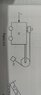# Horizontal force F applied so the relative postion stays constant

• Ithilrandir
In summary, the conversation discusses a problem involving two masses, M and m1, connected by a string with a third mass, m2, hanging from the string. After going through a series of calculations, the correct answer of 392N for the force F is determined to be caused by a fictitious force acting on m2 and the tension in the string. The final equation used to solve for F is T=m1a, where T is the tension in the string, m1 is the mass of one of the objects, and a is the acceleration of the system.f

#### Ithilrandir

Homework Statement
Referring to Fig. 5-4: What horizontal force F must be constantly applied to M=21kg so that m1 = 5kg does not move relative to m2 = 4 kg. Neglect friction.
Relevant Equations
...
g=9.81
m2g=m1a,
a=(m2/m1)g=0.8g

F=0.8g x (M+m1+m2)= 0.8g x (21+5+4)= 0.8g x 30= 235.44 N.

The answer key however indicate that the answer is 392N. This made me think that the tilt of m2 has something to do with it, but I can't see how much it will make F larger than the above answer.

#### Attachments

•WhatsApp Image 2021-01-24 at 9.49.06 PM.jpeg
15 KB · Views: 103
Well, if ##F = 0##, then ##m_2## will fall vertically. But, if ##F \ne 0##, then ##m_2## will be pushed to the left relative to the accelerating system. You definitely have to take that into account.

I see. Is it right to say then that m2 will experience a relative leftward acceleration = F/(30kg)?

I see. Is it right to say then that m2 will experience a relative leftward acceleration = F/(30kg)?
That's the acceleration of the CoM of the system, right?

So, what do we add to the usual Newton's laws here?

I don't think I caught your hint. I was thinking of making m1 accelerate left relative to M so that m2 do not move relative to m1, but I'm a little confused at where to go from there.

I don't think I caught your hint.
Have you heard of a fictictious (or inertial) force?

Yes, I have heard of fictitious force, but I don't know how I to apply it properly as I'm doing the questions with only the lecture as reference. The fictitious force acting on m2 is to the left, so I balance the forces acting on m2 from the reference of M, then convert back to the normal frame of reference?

Yes, I have heard of fictitious force, but I don't know how I to apply it properly as I'm doing the questions with only the lecture as reference. The fictitious force acting on m2 is to the left, so I balance the forces acting on m2 from the reference of M, then convert back to the normal frame of reference?
You don't need to go back to the normal reference frame, as you are asked for the case when there is no relative motion. That condition is independent of the reference frame.

I balanced the forces at the string with T being the square root of (m2g) ^2 + m2a, F=(M + m1 + m2)a.

From here, since T and a are unknown, I had no clue how to carry on. I tried to work backwards from the answer but didn't see any connection.

I balanced the forces at the string with T being the square root of (m2g) ^2 + m2a, F=(M + m1 + m2)a.

From here, since T and a are unknown, I had no clue how to carry on. I tried to work backwards from the answer but didn't see any connection.
I hope you mean ##T =\sqrt{(m_2g) ^2 + (m_2a)^2}##.

What about the balance of forces on m1?

I hope you mean ##T =\sqrt{(m_2g) ^2 + (m_2a)^2}##.

What about the balance of forces on m1?

From the reference of M, on m1 it's T to the right and m1g downwards if I'm not mistaken.

From the reference of M, on m1 it's T to the right and m1g downwards if I'm not mistaken.
So what keeps it place on M? Don't forget the fictitious force.

So what keeps it place on M? Don't forget the fictitious force.
The fictitious force is to the left, but that doesn't seem to be the only force holding it.

The fictitious force is to the left, but that doesn't seem to be the only force holding it.
You already identified the tension in the cord. The fictitious force is the only other force with a horizontal component. So what equation can you write?

You already identified the tension in the cord. The fictitious force is the only other force with a horizontal component. So what equation can you write?
I managed to solve it. I had been mixing up the masses in my calculations. T=m1a, solving for a then multiplying a with 30(total mass) gets the correct F of 392N.

I managed to solve it. I had been mixing up the masses in my calculations. T=m1a, solving for a then multiplying a with 30(total mass) gets the correct F of 392N.
Ok!